# Solving Systems of Linear Equations One Step at a Time

February 13, 2013 —Comments Off

In our previous post about expanding Step-by-step solutions, we introduced a revamped equation solver. I’m proud to say that it has now been extended to solve systems of linear equations. In addition, you have four different methods to choose from when looking for a solution! These methods are elimination, substitution, Gaussian elimination, and Cramer’s rule. Let’s look at x + y = 5, xy = 1 to see all four methods in action.

First we look at elimination: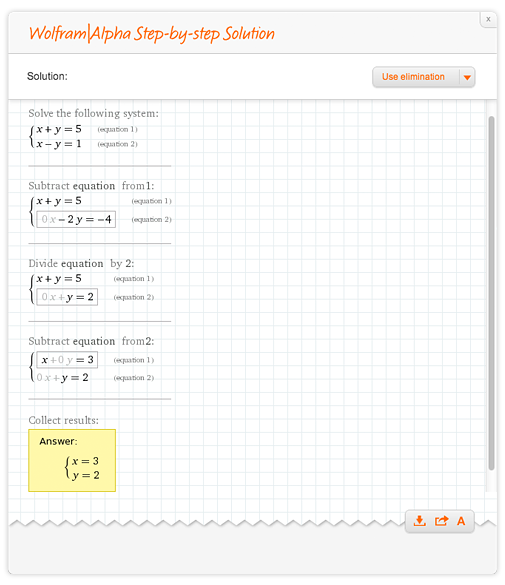Now we solve using substitution: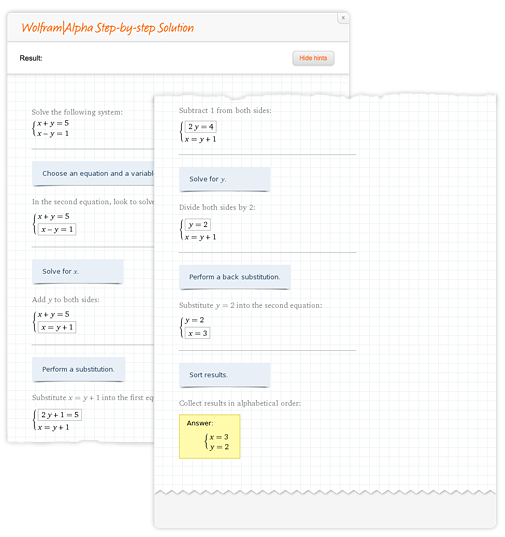Expressing our system in matrix form, we can perform Gaussian elimination: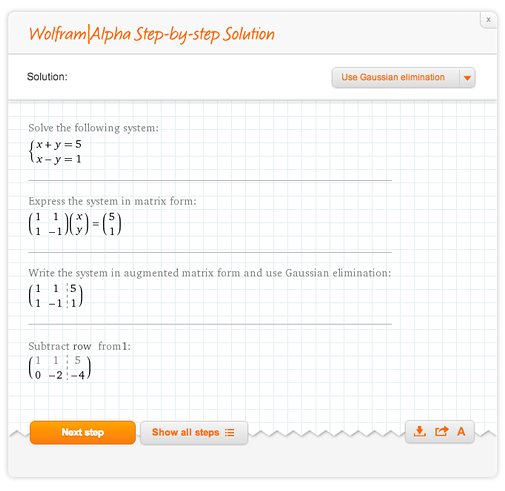Lastly, the determinant can play a fundamental role in solving linear systems. This is called Cramer’s rule: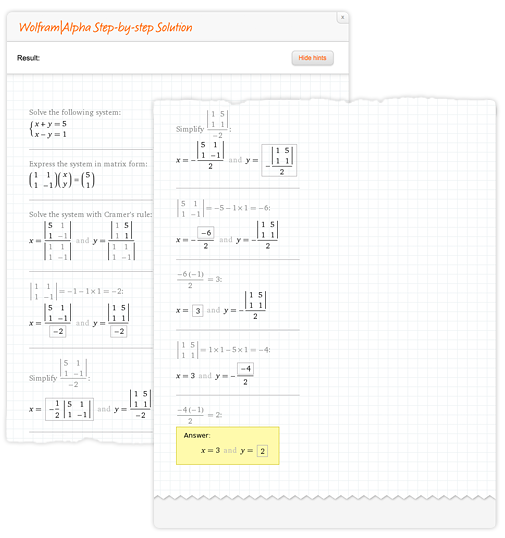The number of unknowns is not a problem for Wolfram|Alpha; here w + x + y + z = 1, 2wx + yz = 0, w + 3x + y + z = 5, w + xy + 2z = 2 is an example of a 4×4 linear system. We have even added this new functionality when solving word problems.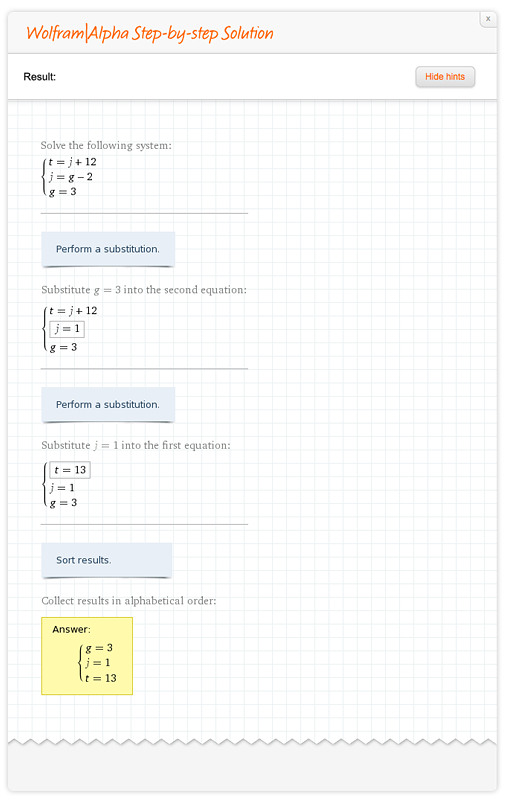Having various methods to choose from is a great way to look at a problem from all angles. Stay tuned for more and more step-by-step math in the future!

Can this be used to solve systems of DE’s?

Posted by shane toreki March 20, 2013 at 11:12 pm

How to solve this system?
3x-2y+z=1;
x+3y-z=2;
x-8y+3z=-3;

Or this one?
3x-2y+z-2w-u=3,
-9x+6y-3z+6w+10u=-2,
3x-y+3z-w-u=4,

Above systems have parametric solutions. But wolfram don’t say which variables are parametric and which are static.
This systems you can solve with matrix, so step by step solution by Gauss elimination would be nice.

Posted by Joe March 19, 2014 at 6:15 pm

did not help at all maybe adding a calculator to solve will help1

Posted by billy joe September 15, 2014 at 3:30 pm

For the initial value problem

y’ = xy^3 / ?1+x^2 y (0) = 1

(a) Find the solution in the explicit form
(b) Plot the graph of the solution
(c) Determine (at least approximately) the interval in which the solution is defined.

Posted by enes kalay November 3, 2014 at 7:44 am

The subsidiary equations are in the form dx/P = dy/P = dz/Q

We have dx dy dz
____ = ____ = ____
x^2-y^2-z^2 2xy 2xz

Using multipliers x, y, z, we have

each fraction= xdx+ydy+zdz
__________
x(x^2+y^2+z^2)

Can you explain how?

Posted by Priti Ravindran February 25, 2015 at 6:05 am

I am trying to solve the system of equations: x^3+3y^2+1=4z, y^3+3z^2+1=4x, z^3+3x^2+1=4y, but cannot find a widget that will do so and show me the steps to understand it. Can anyone help??

Posted by Nicole July 27, 2015 at 8:46 pm